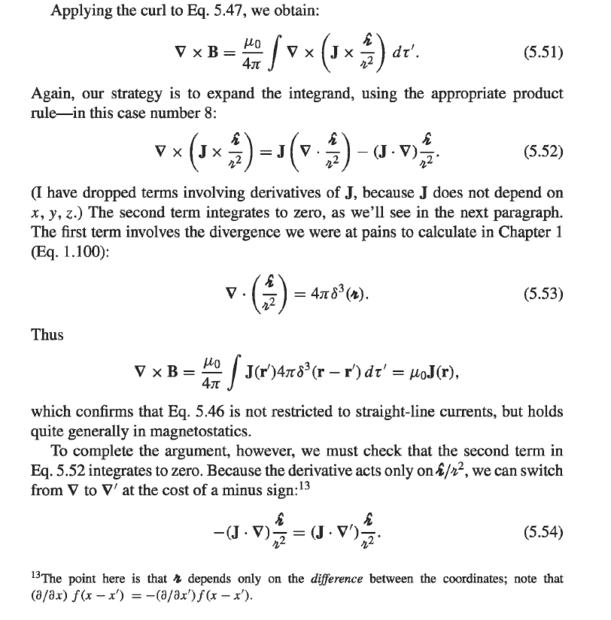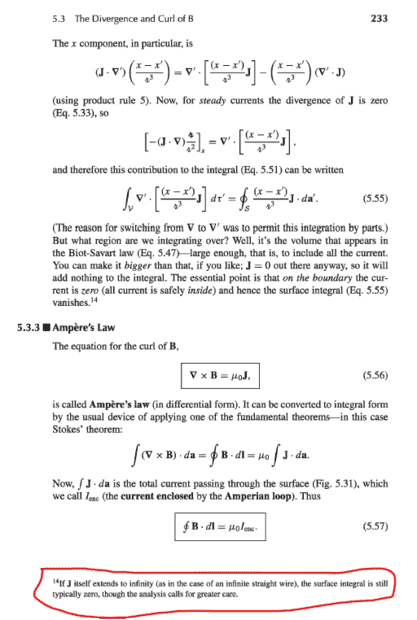# A math confusion in deriving the curl of magnetic field from Biot-Savart

• I
• Brian Tsai

#### Brian Tsai

TL;DR Summary
Why the surface integral is 0 even the J itself extends to infinity (as in the case of an infinite straight wire).
I am recently reading "Introduction to Electrodynamics, Forth Edition, David J. Griffiths " and have a problem with the derive of the curl of a magnetic field from Biot-Savart law. The images of pages (p.232~p233) are in the following:The second term in 5.55(page 233) is 0. I had known the reason in case of that the current density declined to 0 on the surface. My question is how to prove the surface integral will also be 0 when J extends to infinite(red block).

P.S. : This is my first time asking a question in English, and I had done my best to decrease the improper use of English. I sincerely hope that anyone who notices my post can answer my confusion and don't be mad at my terrible use in English

•weirdoguy
. A:In the general case of an arbitrary current density J that extends to infinity, the surface integral in (1) will not be zero.In the special case of a current density that is zero on the surface, then the surface integral will be zero.In the general case, you can think of the surface integral as the sum of contributions from each infinitesimal point on the surface. The electric field due to each such point is given by the Biot-Savart law. If the current density vanishes on the surface, then the contributions from each infinitesimal point will cancel out, and the total electric field on the surface will be zero. This implies that the surface integral will be zero.